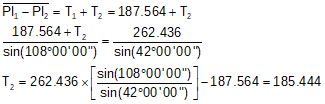### 2. Compound curve

#### a. Nomenclature

Starting with two tangent lines intersecting at a PI, a compound curve is fit between them, Figure D-9.Figure D-9 Compound curve

Figure D-10 shows major parts of the compound curve.Figure D-10 Compound curve parts

The PCC (Point of Curve to Curve) is the EC of the first curve and BC of the second. A tangent line at the PCC intersects the incoming tangent at the PI1 and the outgoing tangent at PI2.

Ti is the distance from the BC1 to the PI; To the distance from the PI to the EC2.

There are seven parts to a compound curve: Ti, To, R1, R2, Δ1, Δ2, and Δ. Because the tangents deflect Δ at the PI and the compound curve transitions from the incoming to the outgoing tangent:

 Δ = Δ1+ Δ2 Equation D-1

That means there are six independent parts. For a unique solution four parts must be fixed, including at least one angle and at least two distances.

Because they share a point (PCC) and tangent line, once all six parts are determined, the compound curve can be computed as two simple curves, Figure D-11. Individual curve attributes can be computed using the single curve equations in Chapter C.Figure D-11 Two simple curves

Depending on which parts are initially fixed, there are different ways to compute the remaining parts.

One way is solving the vertex triangle, Figure D-12, created by the three PIs.Figure D-12 Vertex triangle

Another method is to use a closed loop traverse through points O1-BC1-PI-EC2-O2, Figure D-13.Figure D-13 Traverse method

The direction of one line is assumed to be in a cardinal direction and the others written in terms of 90° angles and Δs. Distances are Rs and Ts. Latitudes and departures are computed, summed, are set eqaul to zero to force closure. This results in two equations in two unknowns which can then be simultaneously solved.

#### b. Examples

##### (1) Example 1

A PI is located at station 38+00.00 with a left deflection of 72°00'00"L. The compound curve begins at sta 33+50.00. The first curve has a 700.00 ft radius and 30°00'00" central angle.

Determine the radius and central angle of the second curve and the length of both curves.

Sketch:We'll try a vertex triangle solution. Isolate the triangle and label the tangents:Since we have the radius and central angle of the first curve, we can compute its tangent:Compute Δ2 using Equation D-1Determine the distance from the PI1 to the PI which is a side of the triangle.The distance between PI1 and PI2 is the sum of the curve tangents. Using the Law of Sines and the known T1, we can compute T2.Using T2 and Δ2, R2 can be determined.Finally, compute each curve's length.##### (2) Example 2

A PI is located at 55+69.23 with a deflection angle between tangents of 85°00'00"R. The compound curve must begin 463.64 ft before the PI and end 405.60 ft after. Central angles of the two curves are 30°00'00" and 55°00'00" respectivley.

Determine the radius of each curve,

Sketch:Because we only have angles of the vertex triangle with no distances (and insufficient given data to compute any), the curve system can't be solved that way. Instead, we will use the traverse method.

To start, the curve system is rotated to make the initial radial line run North. Then using right angles and Δs, bearings of the other lines can be determined.

Updated sketch with bearings:Compute Latitudes and Departures:

 Line Bearing Length Latitude Departure O1-BC1 North R1 R1 0.00 BC1-PI East 463.64 0.00 463.64 PI-EC2 S 5°00'00"E 405.60 -405.60 x cos(5°00'00") +405.60 x sin(5°00'00") EC2-O2 S 85°00'00"W R2 -R2 x cos(85°00'00") -R2 x sin(85°00'00") O2-O1 S 30°00'00"W R1-R2 -(R1-R2) x cos(30°00'00") -(R1-R2) x sin(30°00'00")

Sum and reduce the Latitudes:Sum and reduce the Departures:We have two equatiosn with unkowns R1 and R2. Solve them simultaneously. We'll use substitution.

Solve the Latitide summation for R1Substiute the equation for R1 in the Departures summation and solve R2:Solve R1:With R1 and R2 computed, we have two geometric attributes for each curve. Using Chapter C equations, their remaining attributes can be determined.

#### c. Stationing

As with a single curve, there are two paths to the EC from the BC. One goes up and down the original tangents, the other along the curves. Whether a station equation is used at the EC depends on how the entire alignment is stationed. Refer to the discussion in Chapter C Section 2.

Equations to compute the curves' endpoints are:Equation D-2Equation D-3Equation D-4Equation D-5

The stationing for Example (2)  is:Each curve's table is computed as described in Chapter C.

Following are radial chord tables for each curve in Example (2) (computations aren't shown).

 Curve 1 Sta Defl ang Chord EC 54+10.64 15º00'00" 301.58 54+00 14º28'36" 291.29 53+00 9º33'34" 193.51 52+00 4º38'32" 94.31 BC 51+05.59 0º00'00" 0.00

 Curve 2 Sta Defl ang Chord EC 58+12.43 27º30'00" 386.54 58+00 26º38'57" 375.47 57+00 19º48'17" 283.63 56+00 12º57'37" 187.77 55+00 6º06'57" 89.109 BC 54+10.64 0º00'00" 0.00

Stakeout procedure

Measure Ti and To from the PI along the tangents to set the BC1 and EC2.

Stake Curve 1

Setup instrument on BC1.

Sight PI with 0º00'00" on the horizontal circle.

Stake curve points using the curve table.

Stake Curve 2

Setup on PCC

Sight BC1 with -(Δ1/2) = -15º00'00"=345°00'00" on the horizontal circle, Figure D-14a.

Inverse the scope to sight away from the BC1, Figure D-14b.

Rotating right to a horizontal angle of 0º00'00" orients intrument tangent to the curves at the PCC, Figure D14c.

Stake curve points using the curve table.

Last point should match the previously set EC2.a. Sight BC1 with  -(Δ1/2) readingb. Inverse telescopec. Rotate to 00°00'00" Figure D-14 Orienting at PCC